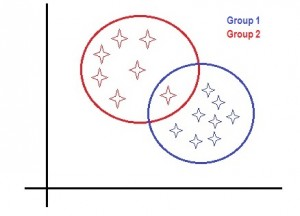# Logistic Regression (Logit Model): a Brief Overview

Share on

Probability and Statistics > Regression Analysis > Logistic Regression / Logit Model

In order to understand logistic regression (also called the logit model), you may find it helpful to review these topics:
The Nominal Scale.
What is Linear Regression?

For a brief look, see: Logistic Regression in one picture.

## Simple Logistic Regression

Simple logistic regression is almost identical to linear regression. However, linear regression uses two measurements and logistic regression uses one measurement and one nominal variable. The measurement variable is always the independent variable. It’s used when you want to find the probability of getting a certain nominal variable when you have a particular measurement variable.

## Logistic Regression, ANOVA and Student’s T-Tests

ANOVA and Student’s T-Tests can also be used to analyze data that has one nominal variable and one measurement variable. Logistic regression is used when you want to predict the probability for the nominal variable. Here’s an example to clarify that statement:

You measure the BMI for a group of 50-year-old women, then ten years later you survey the women to see who had a myocardial infarction (a heart attack). You could evaluate your data in different ways, depending on your goal:

• Student’s T-Test: You can test the null hypothesis that BMI is not linked to myocardial infarction.
• Logistic Regression: You can predict the probability that a 50-year-old woman with a certain BMI would have a heart attack in the next decade.

## Logistic Regression vs. Linear Regression

In linear regression, you must have two measurements (x and y). In logistic regression, your dependent variable (your y variable) is nominal. In the above example, your y variable could be “had a myocardial infarction” vs. “did not have a myocardial infarction.” However, you can’t plot those nominal variables on a graph, so what you do is plot the probability of each variable (from 0 to 1). For example, your study might show that a woman with a BMI of 30 has a 4% chance of having a heart attack within the next ten years; you could plot that as 30 for the X variable and 0.04 for the Y variable.

## Comparison with Discriminant Analysis

Discriminant analysis is a classification method that gets its name from discriminating, the act of recognizing a difference between certain characteristics. The two goals are:

• Construction of a classification method to separate members of a population.
• Using the classification method to allocate new members to groups within the population.Discriminant Analysis is used when you have a set of naturally formed groups and you want to find out which continuous variables discriminate between them. The simplest example of DA is to use a single variable to predict where a member will fall in a population. For example, using high school GPA to predict whether a student will drop out of college, graduate from college, or graduate with honors.

A more complex example: you might want to find out which variables discriminate between credit applicants who are a high, medium, or low risk for default. You could collect data on credit card holder characteristics and use that information to determine what variables are the best predictors for whether a particular person will be a high, medium, or low risk. New observations (in this case, new applicants) could then be allocated to a particular group.

As well as the credit and banking industries, other uses for Discriminant Analysis include:

Logistic Regression is often preferred over Discriminant Analysis as it can handle categorical variables and continuous variables. Logistic Regression also does not have as many assumptions associated with it. For example, Discriminant Analysis requires the assumptions of equal variance-covariance within each group, multivariate normality, and the data must be linearly related. Logistic Regression does not have these requirements.

## Related Articles

Check out our YouTube channel for hundreds of videos on elementary statistics and probability.

CITE THIS AS:
Stephanie Glen. "Logistic Regression (Logit Model): a Brief Overview" From StatisticsHowTo.com: Elementary Statistics for the rest of us! https://www.statisticshowto.com/logistic-regression/
---------------------------------------------------------------------------Need help with a homework or test question? With Chegg Study, you can get step-by-step solutions to your questions from an expert in the field. Your first 30 minutes with a Chegg tutor is free!